Publicité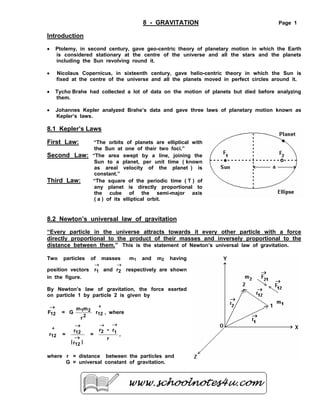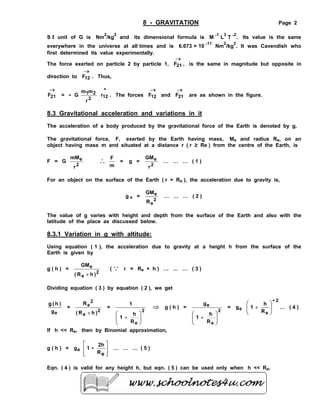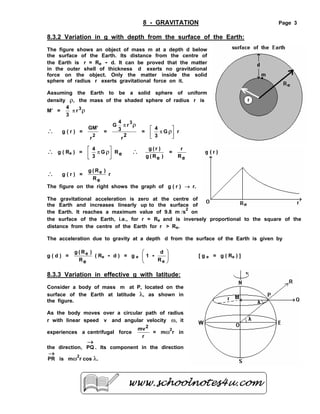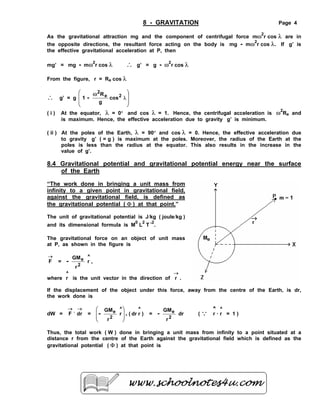Publicité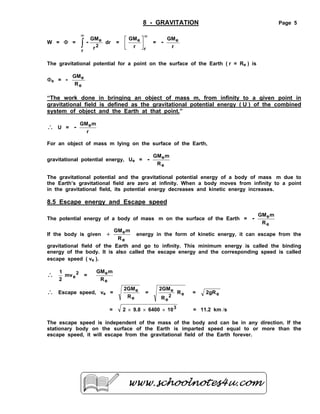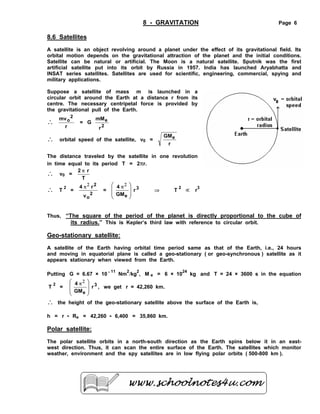Prochain SlideShare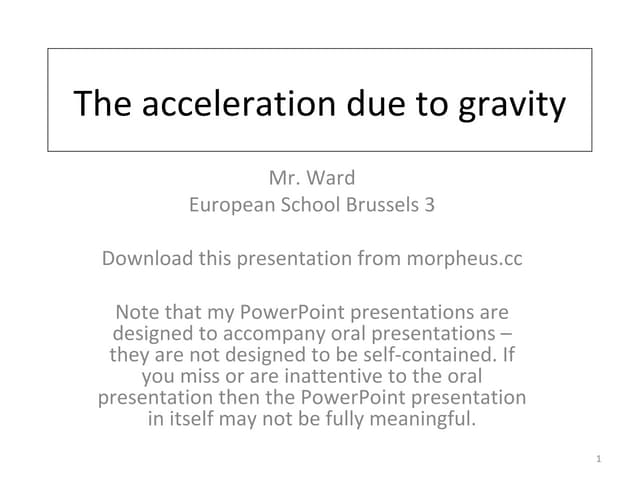Acceleration due to gravity
Chargement dans ... 3
1 sur 6
Publicité

### 08 gravitation

1. 8 - GRAVITATION Page 1 Introduction • Ptolemy, in second century, gave geo-centric theory of planetary motion in which the Earth is considered stationary at the centre of the universe and all the stars and the planets including the Sun revolving round it. • Nicolaus Copernicus, in sixteenth century, gave helio-centric theory in which the Sun is fixed at the centre of the universe and all the planets moved in perfect circles around it. • Tycho Brahe had collected a lot of data on the motion of planets but died before analyzing them. • Johannes Kepler analyzed Brahe’s data and gave three laws of planetary motion known as Kepler’s laws. 8.1 Kepler’s Laws First Law: “The orbits of planets are elliptical with the Sun at one of their two foci.” Second Law: “The area swept by a line, joining the Sun to a planet, per unit time ( known as areal velocity of the planet ) is constant.” Third Law: “The square of the periodic time ( T ) of any planet is directly proportional to the cube of the semi-major axis ( a ) of its elliptical orbit. 8.2 Newton’s universal law of gravitation “Every particle in the universe attracts towards it every other particle with a force directly proportional to the product of their masses and inversely proportional to the distance between them.” This is the statement of Newton’s universal law of gravitation. Two particles of masses m1 and m2 having position vectors → 1r and → 2r respectively are shown in the figure. By Newton’s law of gravitation, the force exerted on particle 1 by particle 2 is given by → 12F = G ^ 122 21 r r mm , where ^ 12r = r r 12 12 ll → → = r rr 12 - →→ , where r = distance between the particles and G = universal constant of gravitation.
2. 8 - GRAVITATION Page 2 S I unit of G is Nm 2 /kg 2 and its dimensional formula is M -1 L 3 T -2 . Its value is the same everywhere in the universe at all times and is 6.673 × 10 -11 Nm 2 /kg 2 . It was Cavendish who first determined its value experimentally. The force exerted on particle 2 by particle 1, → 21F , is the same in magnitude but opposite in direction to → 12F . Thus, → 21F = - G ^ 122 21 r r mm . The forces → 12F and → 21F are as shown in the figure. 8.3 Gravitational acceleration and variations in it The acceleration of a body produced by the gravitational force of the Earth is denoted by g. The gravitational force, F, exerted by the Earth having mass, Me and radius Re, on an object having mass m and situated at a distance r ( r ≥ Re ) from the centre of the Earth, is F = G 2 e r mM ∴ m F = g = 2 e r GM … … … ( 1 ) For an object on the surface of the Earth ( r = Re ), the acceleration due to gravity is, g e = 2 e e R GM … … … ( 2 ) The value of g varies with height and depth from the surface of the Earth and also with the latitude of the place as discussed below. 8.3.1 Variation in g with altitude: Using equation ( 1 ), the acceleration due to gravity at a height h from the surface of the Earth is given by g ( h ) = 2 e e )hR( GM + ( Q r = Re + h ) … … … ( 3 ) Dividing equation ( 3 ) by equation ( 2 ), we get eg )h(g = 2 e 2 e )hR( R + = 2 eR h 1 1         + ⇒ g ( h ) = 2 e e R h 1 g         + = eg 2 e - R h 1         + … ( 4 ) If h << Re, then by Binomial approximation, g ( h ) = eg         eR 2h 1 - … … … ( 5 ) Eqn. ( 4 ) is valid for any height h, but eqn. ( 5 ) can be used only when h << Re.
3. 8 - GRAVITATION Page 3 8.3.2 Variation in g with depth from the surface of the Earth: The figure shows an object of mass m at a depth d below the surface of the Earth. Its distance from the centre of the Earth is r = Re - d. It can be proved that the matter in the outer shell of thickness d exerts no gravitational force on the object. Only the matter inside the solid sphere of radius r exerts gravitational force on it. Assuming the Earth to be a solid sphere of uniform density ρ, the mass of the shaded sphere of radius r is M’ = ρπ 3r 3 4 ∴ g ( r ) = 2 r GM' = 2 r r 3 4 G 3 ρπ = rG 3 4       π ρ ∴ g ( Re ) = eRG 3 4       π ρ ∴ )eR(g )r(g = eR r ∴ g ( r ) = r eR )R(g e The figure on the right shows the graph of g ( r ) → r. The gravitational acceleration is zero at the centre of the Earth and increases linearly up to the surface of the Earth. It reaches a maximum value of 9.8 m /s 2 on the surface of the Earth, i.e., for r = Re and is inversely proportional to the square of the distance from the centre of the Earth for r > Re. The acceleration due to gravity at a depth d from the surface of the Earth is given by g ( d ) = eR )R(g e ( Re - d ) = g e         eR d 1 - [ g e = g ( Re ) ] 8.3.3 Variation in effective g with latitude: Consider a body of mass m at P, located on the surface of the Earth at latitude λ, as shown in the figure. As the body moves over a circular path of radius r with linear speed v and angular velocity ω, it experiences a centrifugal force r mv2 = mω2 r in the direction, → PQ . Its component in the direction → PR is mω2 r cos λ.
4. 8 - GRAVITATION Page 4 As the gravitational attraction mg and the component of centrifugal force mω2 r cos λ are in the opposite directions, the resultant force acting on the body is mg - mω2 r cos λ. If g’ is the effective gravitational acceleration at P, then mg’ = mg - mω2 r cos λ ∴ g’ = g - ω2 r cos λ From the figure, r = Re cos λ ∴ g’ = g         λ ω cos g R 1 2e 2 - ( i ) At the equator, λ = 0° and cos λ = 1. Hence, the centrifugal acceleration is ω2 Re and is maximum. Hence, the effective acceleration due to gravity g’ is minimum. ( ii ) At the poles of the Earth, λ = 90° and cos λ = 0. Hence, the effective acceleration due to gravity g’ ( = g ) is maximum at the poles. Moreover, the radius of the Earth at the poles is less than the radius at the equator. This also results in the increase in the value of g’. 8.4 Gravitational potential and gravitational potential energy near the surface of the Earth “The work done in bringing a unit mass from infinity to a given point in gravitational field, against the gravitational field, is defined as the gravitational potential ( Φ ) at that point.” The unit of gravitational potential is J/kg ( joule/kg ) and its dimensional formula is M 0 L 2 T -2 . The gravitational force on an object of unit mass at P, as shown in the figure is → F = ^ 2 e r r GM - , where ^ r is the unit vector in the direction of → r . If the displacement of the object under this force, away from the centre of the Earth, is dr, the work done is dW = → F . → dr = )rdr(r r GM ^^ 2 e .-         = 2 e r GM - dr ( Q ^ r ^ r ⋅ = 1 ) Thus, the total work ( W ) done in bringing a unit mass from infinity to a point situated at a distance r from the centre of the Earth against the gravitational field which is defined as the gravitational potential ( Φ ) at that point is
5. 8 - GRAVITATION Page 5 W = Φ = dr r GM r 2 e -∫ ∞ = ∞       r e r GM = r GMe- The gravitational potential for a point on the surface of the Earth ( r = Re ) is Φe = - e e R GM “The work done in bringing an object of mass m, from infinity to a given point in gravitational field is defined as the gravitational potential energy ( U ) of the combined system of object and the Earth at that point.” ∴ U = r mGMe- For an object of mass m lying on the surface of the Earth, gravitational potential energy, Ue = e e R mGM - The gravitational potential and the gravitational potential energy of a body of mass m due to the Earth’s gravitational field are zero at infinity. When a body moves from infinity to a point in the gravitational field, its potential energy decreases and kinetic energy increases. 8.5 Escape energy and Escape speed The potential energy of a body of mass m on the surface of the Earth = e e R mGM - If the body is given e e R mGM + energy in the form of kinetic energy, it can escape from the gravitational field of the Earth and go to infinity. This minimum energy is called the binding energy of the body. It is also called the escape energy and the corresponding speed is called escape speed ( ve ). ∴ 2 emv 2 1 = e e R mGM ∴ Escape speed, ve = e e R 2GM = R R 2GM e2 e e = e2gR = 31064009.82 ××× = 11.2 km /s The escape speed is independent of the mass of the body and can be in any direction. If the stationary body on the surface of the Earth is imparted speed equal to or more than the escape speed, it will escape from the gravitational field of the Earth forever.
6. 8 - GRAVITATION Page 6 8.6 Satellites A satellite is an object revolving around a planet under the effect of its gravitational field. Its orbital motion depends on the gravitational attraction of the planet and the initial conditions. Satellite can be natural or artificial. The Moon is a natural satellite. Sputnik was the first artificial satellite put into its orbit by Russia in 1957. India has launched Aryabhatta and INSAT series satellites. Satellites are used for scientific, engineering, commercial, spying and military applications. Suppose a satellite of mass m is launched in a circular orbit around the Earth at a distance r from its centre. The necessary centripetal force is provided by the gravitational pull of the Earth. ∴ r mv 2 o = G 2 e r mM ∴ orbital speed of the satellite, v0 = r GMe The distance traveled by the satellite in one revolution in time equal to its period T = 2πr. ∴ v0 = T r2 π ∴ T 2 = 2 o 2 v r4 2π = 3 e r GM 4         π2 ⇒ T 2 ∝ r 3 Thus, “The square of the period of the planet is directly proportional to the cube of its radius.” This is Kepler’s third law with reference to circular orbit. Geo-stationary satellite: A satellite of the Earth having orbital time period same as that of the Earth, i.e., 24 hours and moving in equatorial plane is called a geo-stationary ( or geo-synchronous ) satellite as it appears stationary when viewed from the Earth. Putting G = 6.67 × 10 - 11 Nm 2 /kg 2 , M e = 6 × 10 24 kg and T = 24 × 3600 s in the equation T 2 = 3 e r GM 4         π2 , we get r = 42,260 km. ∴ the height of the geo-stationary satellite above the surface of the Earth is, h = r - Re = 42,260 - 6,400 = 35,860 km. Polar satellite: The polar satellite orbits in a north-south direction as the Earth spins below it in an east- west direction. Thus, it can scan the entire surface of the Earth. The satellites which monitor weather, environment and the spy satellites are in low flying polar orbits ( 500-800 km ).
Publicité